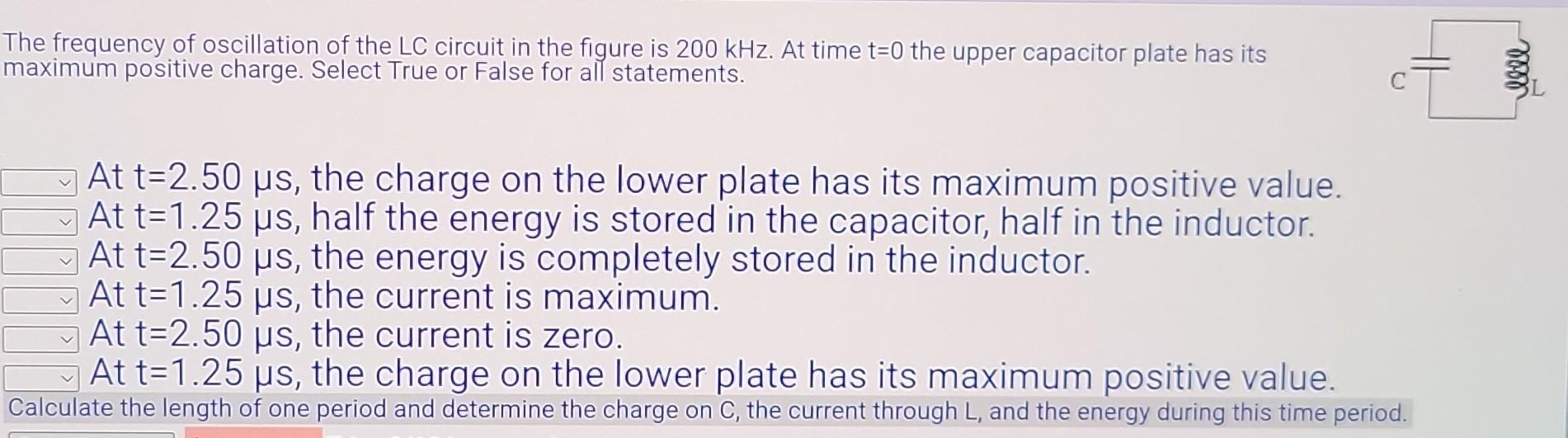Home / Expert Answers / Physics / the-frequency-of-oscillation-of-the-lc-circuit-in-the-figure-is-200khz-at-time-t-0-the-upper-c-pa571

# (Solved): The frequency of oscillation of the LC circuit in the figure is 200kHz. At time t=0 the upper c ...The frequency of oscillation of the LC circuit in the figure is . At time the upper capacitor plate has its naximum positive charge. Select True or False for all statements. At , the charge on the lower plate has its maximum positive value. At , half the energy is stored in the capacitor, half in the inductor. At , the energy is completely stored in the inductor. At , the current is maximum. At , the current is zero. At , the charge on the lower plate has its maximum positive value. Calculate the length of one period and determine the charge on C, the current through , and the energy during this time period.

We have an Answer from Expert